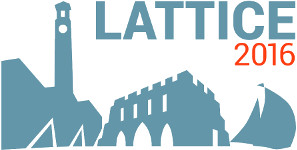#The 34th International Symposium on Lattice Field Theory (Lattice 2016)

Jul 24 – 30, 2016
Highfield Campus, University of Southampton
Europe/London timezone

## The coupled channel approach to the Lambda_c N - Sigma_c N system in lattice QCD

Jul 28, 2016, 5:50 PM
20m
Building B2a Room 2065 (Highfield Campus, University of Southampton)

### Speaker

Mr Takaya for HAL QCD Collaboration Miyamoto (Yukawa Institute for Theoretical Physics, Kyoto University)

### Description

We investigate the 2-baryon system with the isospin 1/2 including one charm quark, namely, $\Lambda_c N$ and $\Sigma_c N$. Although this system is similar to the $\Lambda N$ and $\Sigma N$ system, the interaction of this charmed 2-baryon system above $\Sigma_c N$ threshold might be quite different from its hyperon counter part, due to the smaller mass splitting between $\Sigma_c$ and $\Sigma_c^*$ than $\Sigma$ and $\Sigma^*$, which is consequence of the heavy quark spin symmetry. To explore this possibility, we calculate the coupled channel potential for charmed 2-baryon system using the HAL QCD method in lattice QCD. To avoid the discretization error coming from the heavy quark mass, we employ the Relativistic Heavy Quark (RHQ) action for the charm quark. The purpose of this talk is to present our latest results and to discuss the nature of the interaction in the charmed 2-baryon system including a possibility on the existence of bound or resonance states.

### Primary author

Mr Takaya for HAL QCD Collaboration Miyamoto (Yukawa Institute for Theoretical Physics, Kyoto University)

 Slides